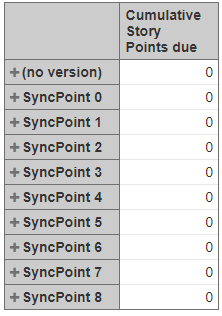# Fix Version rank does not seem to work

Hello,
I’m trying to get a simple table where I have the [fix versions] dimension as a row and [measures].[story points due] as column.
I’m now trying to create a cumulative [measures].[cumulative story points due] with the following formula:
Sum(
childrenset([Fix Version].[All Fix Versions]),
Rank([Fix Version].CurrentMember,
ChildrenSet([Fix Version].[All Fix Versions])
)
), [Measures].[Story Points due]
)
This gives me an empty column.

Investigating further I would expect that a formula like:
Rank([Fix Version].CurrentMember,
ChildrenSet([Fix Version].[All Fix Versions])
)
would return something like 1,2, 3, 4, etc… for each fix version. Nevertheless it returns 0 for all rows.

I have based my formula on the one I found here:
https://eazybi.com/accounts/1632/cubes/Issues/reports/36491-example-cumulative-bugs-created-in-fix-versions

I’m using eazyBI add-on for JIRA version 4.3.2.

What am I doing wrong?

Hi

ChildrenSet from Default member in Fix version dimension will retrieve members of the first level only. You would like to get a set of version member to rank them and use in cumulative caluclation.

``````Sum(
[Fix Version].[Version].Members,
[Fix version].CurrentMember.Name <> '(no version)'),
Rank(
[Fix Version].CurrentMember,
Filter(
[Fix Version].[Version].Members,
[Fix version].CurrentMember.Name <> '(no version)')
)),  [Measures].[Story Points due]
)
``````

Childrenset is useful if you are working with some calculated memmber. For example, if you have a calculated member in Fix version dimension aggregating Versions, you can use the formula to retrieve all versions members from this calculated member with this function :

``````ChildrenSet([Fix version].[Calcuated member over versions]).
``````

Hello Daina,
Thanks for your reply. I’ve changed the formula but it continues to give me empty cells in the table.
To figure out the reason I commented everything so that only the Rank() function remains:
Rank(
[Fix Version].CurrentMember,
Filter(
[Fix Version].[Version].Members,
[Fix version].CurrentMember.Name <> ‘(no version)’)
)
This gives me the following table:(the SyncPoints are the fix versions)

Instead of showing zeros, shouldn’t this column show 1,2,3,4,…?

Summary

This text will be hidden

Hi Jacques

Thanks for additional details. It seems you are using no default hierarchy, but versions by name on version level.

I updated the formula to get it working for any Fix version hierarchy at selected level:

``````Sum(
[Fix Version].CurrentHierarchy.Level.Members,
[Fix version].CurrentHierarchyMember.Name <> '(no version)'),
Rank(
[Fix Version].CurrentHierarchyMember,
Filter(
[Fix Version].CurrentHierarchy.Level.Members,
[Fix version].CurrentHierarchyMember.Name <> '(no version)'))
),  [Measures].[Story Points due]
)
``````

hi Daina,

This last formula did the job!
Great work!

ThanksJacques.